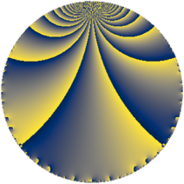# Properties

 Label 121.4.cLevel $121$ Weight $4$ Character orbit 121.c Rep. character $\chi_{121}(3,\cdot)$ Character field $\Q(\zeta_{5})$ Dimension $92$ Newform subspaces $10$ Sturm bound $44$ Trace bound $3$

# Related objects

## Defining parameters

 Level: $$N$$ $$=$$ $$121 = 11^{2}$$ Weight: $$k$$ $$=$$ $$4$$ Character orbit: $$[\chi]$$ $$=$$ 121.c (of order $$5$$ and degree $$4$$) Character conductor: $$\operatorname{cond}(\chi)$$ $$=$$ $$11$$ Character field: $$\Q(\zeta_{5})$$ Newform subspaces: $$10$$ Sturm bound: $$44$$ Trace bound: $$3$$ Distinguishing $$T_p$$: $$2$$

## Dimensions

The following table gives the dimensions of various subspaces of $$M_{4}(121, [\chi])$$.

Total New Old
Modular forms 156 124 32
Cusp forms 108 92 16
Eisenstein series 48 32 16

## Trace form

 $$92 q + 7 q^{2} + 5 q^{3} - 83 q^{4} + 3 q^{5} + 29 q^{6} + 35 q^{7} - 47 q^{8} - 182 q^{9} + O(q^{10})$$ $$92 q + 7 q^{2} + 5 q^{3} - 83 q^{4} + 3 q^{5} + 29 q^{6} + 35 q^{7} - 47 q^{8} - 182 q^{9} - 40 q^{10} - 358 q^{12} + 65 q^{13} + 248 q^{14} + 83 q^{15} + 245 q^{16} + 31 q^{17} + 102 q^{18} - 148 q^{19} - 426 q^{20} - 334 q^{21} + 52 q^{23} + 447 q^{24} + 148 q^{25} + 148 q^{26} - 166 q^{27} + 42 q^{28} + 199 q^{29} + 114 q^{30} + 369 q^{31} - 324 q^{32} + 530 q^{34} - 237 q^{35} - 26 q^{36} - 33 q^{37} + 290 q^{38} - 365 q^{39} - 532 q^{40} + 31 q^{41} + 294 q^{42} + 650 q^{43} - 1204 q^{45} - 1204 q^{46} - 1259 q^{47} - 254 q^{48} - 1110 q^{49} + 147 q^{50} + 246 q^{51} + 590 q^{52} + 1719 q^{53} + 3100 q^{54} + 2664 q^{56} - 102 q^{57} - 1048 q^{58} - 478 q^{59} - 400 q^{60} + 525 q^{61} - 2456 q^{62} + 68 q^{63} + 861 q^{64} - 1790 q^{65} - 806 q^{67} - 710 q^{68} - 228 q^{69} + 288 q^{70} - 1027 q^{71} - 919 q^{72} + 2155 q^{73} + 1476 q^{74} + 54 q^{75} + 242 q^{76} + 2716 q^{78} + 861 q^{79} + 3156 q^{80} + 1489 q^{81} + 3915 q^{82} - 52 q^{83} + 84 q^{84} - 2383 q^{85} - 1203 q^{86} - 2310 q^{87} - 5382 q^{89} + 1682 q^{90} - 3871 q^{91} + 1158 q^{92} - 2013 q^{93} - 702 q^{94} + 1317 q^{95} - 1252 q^{96} - 2094 q^{97} - 2740 q^{98} + O(q^{100})$$

## Decomposition of $$S_{4}^{\mathrm{new}}(121, [\chi])$$ into newform subspaces

Label Dim $A$ Field CM Traces $q$-expansion
$a_{2}$ $a_{3}$ $a_{5}$ $a_{7}$
121.4.c.a $4$ $7.139$ $$\Q(\zeta_{10})$$ $$\Q(\sqrt{-11})$$ $$0$$ $$-8$$ $$-18$$ $$0$$ $$q+8\zeta_{10}^{2}q^{3}+8\zeta_{10}^{3}q^{4}-18\zeta_{10}q^{5}+\cdots$$
121.4.c.b $8$ $7.139$ 8.0.$$\cdots$$.1 None $$-3$$ $$-3$$ $$18$$ $$-10$$ $$q+(\beta _{4}+\beta _{5}+\beta _{6})q^{2}+(\beta _{1}+\beta _{3}-\beta _{4}+\cdots)q^{3}+\cdots$$
121.4.c.c $8$ $7.139$ 8.0.324000000.3 None $$-2$$ $$2$$ $$-2$$ $$-20$$ $$q+(\beta _{2}+\beta _{7})q^{2}+(-4\beta _{1}-\beta _{6})q^{3}+(-2\beta _{1}+\cdots)q^{4}+\cdots$$
121.4.c.d $8$ $7.139$ 8.0.324000000.3 None $$-2$$ $$8$$ $$10$$ $$-8$$ $$q+(\beta _{2}-\beta _{7})q^{2}+(\beta _{1}-4\beta _{6})q^{3}+(2\beta _{1}+\cdots)q^{4}+\cdots$$
121.4.c.e $8$ $7.139$ 8.0.$$\cdots$$.7 None $$0$$ $$10$$ $$-10$$ $$0$$ $$q+\beta _{7}q^{2}-5\beta _{6}q^{3}+18\beta _{4}q^{4}+(-5+\cdots)q^{5}+\cdots$$
121.4.c.f $8$ $7.139$ 8.0.324000000.3 None $$2$$ $$2$$ $$-2$$ $$20$$ $$q+(-\beta _{2}+\beta _{7})q^{2}+(4\beta _{1}-\beta _{6})q^{3}+(2\beta _{1}+\cdots)q^{4}+\cdots$$
121.4.c.g $8$ $7.139$ 8.0.324000000.3 None $$2$$ $$8$$ $$10$$ $$8$$ $$q+(-\beta _{2}+\beta _{7})q^{2}+(\beta _{1}-4\beta _{6})q^{3}+(2\beta _{1}+\cdots)q^{4}+\cdots$$
121.4.c.h $8$ $7.139$ 8.0.$$\cdots$$.1 None $$3$$ $$-3$$ $$18$$ $$10$$ $$q+(1+2\beta _{4}+\beta _{6}-\beta _{7})q^{2}+(-\beta _{1}+\beta _{2}+\cdots)q^{3}+\cdots$$
121.4.c.i $8$ $7.139$ 8.0.$$\cdots$$.1 None $$7$$ $$-3$$ $$-7$$ $$35$$ $$q+(1-\beta _{1}-\beta _{2}+\beta _{4})q^{2}+(-2-2\beta _{2}+\cdots)q^{3}+\cdots$$
121.4.c.j $24$ $7.139$ None $$0$$ $$-8$$ $$-14$$ $$0$$

## Decomposition of $$S_{4}^{\mathrm{old}}(121, [\chi])$$ into lower level spaces

$$S_{4}^{\mathrm{old}}(121, [\chi]) \cong$$ $$S_{4}^{\mathrm{new}}(11, [\chi])$$$$^{\oplus 2}$$